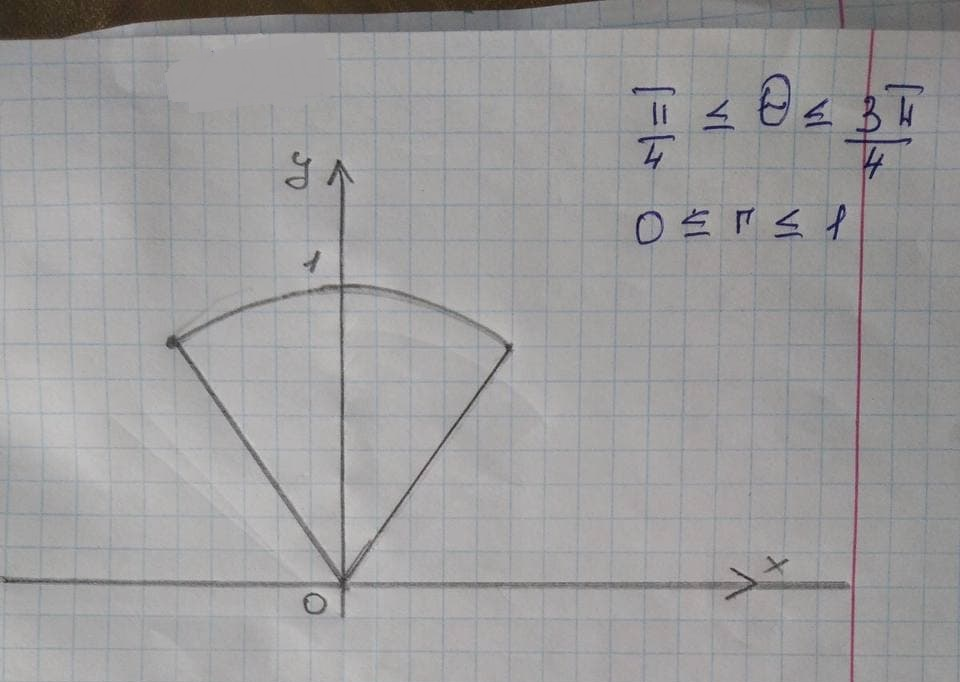# Analyze and sketch a graph of the function. Label anyMahagnazk 2021-11-23 Answered
Analyze and sketch a graph of the function. Label any intercepts, relative extrema, points of inflection, and asymptotes. Use a graphing utility to verify your results $$\displaystyle{y}={3}{x}^{{{\frac{{{2}}}{{{3}}}}}}-{2}{x}$$

• Questions are typically answered in as fast as 30 minutes

### Plainmath recommends

• Get a detailed answer even on the hardest topics.
• Ask an expert for a step-by-step guidance to learn to do it yourself.Luis Sullivan

Step 1 Given Function
$$\displaystyle{y}={3}{x}^{{{\frac{{{2}}}{{{3}}}}}}-{2}{x}$$
To Find: Relative extreme, Point of inflection and Asymptotes.
On Sketching the graph of given function we get,Step 2: From first derivative test definition
Suppose that isa critical point of then
If $$\displaystyle{f}{\left({x}\right)}{>}{0}$$ to the left of $$\displaystyle{x}={c}$$ and $$\displaystyle{f}{\left({x}\right)}{<}{0}$$ to the right of $$\displaystyle{x}={c}$$ then $$\displaystyle{x}={c}$$ is a local maximum.
If $$\displaystyle{f}{\left({x}\right)}{<}{0}$$ to the left of $$\displaystyle{x}={c}$$ and f(x)>0 to the right of $$\displaystyle{x}={c}$$ then $$\displaystyle{x}={c}$$ is a local minimum.
If f(x) is the same sign on both sides of $$\displaystyle{x}={c}$$ then $$\displaystyle{x}={c}$$ is neither a local maximum nor a local local minimum.
Step 3: On differentiating the given equation we obtain,
$$\displaystyle{\frac{{{\left.{d}{y}\right.}}}{{{\left.{d}{x}\right.}}}}={3}\times{\left\lbrace{\frac{{{2}}}{{{3}}}}\right\rbrace}{x}^{{-{\frac{{{1}}}{{{3}}}}}}-{2}$$
$$\displaystyle{\frac{{{\left.{d}{y}\right.}}}{{{\left.{d}{x}\right.}}}}={\left\lbrace{\frac{{{2}}}{{{x}^{{{\frac{{{1}}}{{{3}}}}}}}}}\right\rbrace}-{2}$$
Now, to find critical points substitute,
$$\displaystyle{\frac{{{\left.{d}{y}\right.}}}{{{\left.{d}{x}\right.}}}}={0}$$
$$\displaystyle{\left\lbrace{\frac{{{2}}}{{{x}^{{{\frac{{{1}}}{{{3}}}}}}}}}\right\rbrace}-{2}={0}$$
$$\displaystyle{\left\lbrace{\frac{{{2}}}{{{x}^{{{\frac{{{1}}}{{{3}}}}}}}}}\right\rbrace}={2}$$
$$\displaystyle{x}^{{{\frac{{{1}}}{{{3}}}}}}={1}$$
$$\displaystyle{x}={1}$$
So the critical points obtained
$$\displaystyle{x}={O}$$ and $$\displaystyle{x}={1}$$
$$-\propto$$ So the intervals are
Checking the sign of f(x)
at each monotone interval we have,
Step 5: By the Inflection point Definition
An inflection point is a point on graph at which the second derivative changes sign
If $$\displaystyle{f}\text{}{\left({x}\right)}{>}{0}$$ then f(x) concave upwards
IF $$\displaystyle{f}\text{}{\left({x}\right)}{<}{0}$$ then f(x) concave downwards
Here, We have,
$$\displaystyle{f}\text{}{\left({x}\right)}=-{\frac{{{2}}}{{{3}{x}^{{\frac{{{4}}}{{{3}}}}}}}}$$
Checking the sign we obtain,
$$\begin{array}{|c|c|}\hline &-\propto<x<0&x=0&0<x<\propto\\ \hline Sign&-&NA&+\\ \hline Behavior&Concave Downward&NA&Concave Downward\\ \hline \end{array}$$

On the Above analysis we find that there are
No any point of Inflection that we have for the given function.
Resulting in No any Vertical as well as Horizontal Asymptotes.